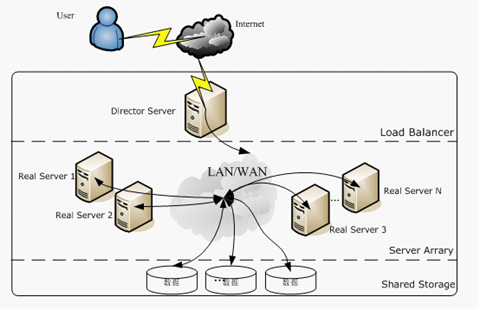# 分层时间记忆算法HTM

## HTM简介

HTM是一种通用人工智慧（或称强人工智慧）的模型。这个模型的背后是基于对脑神经科学的了解、结合数学与电脑科学理论、最终组合起来的一种学习方法，是一种对人类大脑学习能力的模仿。即使最初的想法跟神经网路有些相似之处，但在后来的发展与实作的细节上，走的是另一条完全不一样的路。

HTM算法表面上与神经网络算法有相似之处，其实质是完全不同的。这就好比一般电路与门电路的区别。将模拟“神经元”按照新大脑皮层的结构连接之后就会产生与一般神经网路完全不同的效果。一般的神经网络注重前馈，而HTM算法更注重信息的双向交流，这也是由于神经解剖学发现反馈突触数量不亚于前馈的原因。而反馈并不能得到大多数人的重视。

HTM算法也是一种拥有记忆性和可学习性的算法。它相对于其他学习算法更注重对神经网络的训练而不是架构。HTM算法认为只要经过合理的训练，该算法适用于解决大多数人工智能问题。对于不同的任务目标实验者需要将数据先空间化和时间化再对HTM网络进行训练即可。

HTM包含新大脑皮质的三个显著特性：

• 记忆系统。数据流通过感观进入大脑皮质，其中的神元细胞通建立突触(snapses)连接，达到学习的目的，并将记忆储存其中。每个神经元都可看成一个“记忆”系统。
• 记忆主要为时间（time-changing/temporal)模式。数据在新皮质上的输入输出是实时动态的，而且皮质的对输入数据的学习也是基于时间的，并基于此对动态信息进行预判。
• 层级连接。新皮质内的神经元在皮质内是以层级的方式连接的。因为所有的神经元都执行同样的基本记忆操作，故理解一个神经元的工作方式，即可理解整个皮质的工作方式。

HTM模型相对于现行类神经网路模型的优势：

• 持续学习/在线学习。类神经网路分类器一般在训练完毕、内部权重确定之后，就会切换为predition mode开始正式上线服役。但在现实世界中，新的资料仍会持续形成新的pattern。所以类神经网路模型通常会需要在上线一段时间之后，下线用新的资料重新训练。如何让类神经网路模型能够在服役同时仍保有学习能力，一直仍是大家各说各话，没有统一的最佳答案。HTM则号称能原生保有这样的能力。
• 噪声鲁棒性和容错性。我们目前知道，类神经网路对于输入资料的细微扰动非常敏感。分类错误的资料、遗失的资料、或刻意变造的资料都可以相当大程度的干扰类神经网路的训练与预测能力。如何在类神经网路中增强抵抗杂讯干扰的能力依然是个研究中的问题。但HTM号称有原生的抗噪能力。
• 没有超参数调整。基于类神经网路模型的人工智慧一直被人嘲笑为「炼丹」、机器学习工程师自嘲是「链金术士」或「炼丹师」，就是因为在训练类神经网路模型的过程中有太多的hyperparameter需要决定，但该怎么决定却几乎都凭经验跟运气，甚至有「收敛看运气，效果看天命」的说法。没有一个有系统的、有数学理论支撑的决定方式。最简单的例子，光是Learning rate要怎么调，就有一大堆的paper。光是网路的架构要怎么设定（要几层？用什么层？太少层精确度不高，太多层又会梯度消失直接在训练阶段爆炸）就可以搞死人。即使后来有NAS与自动语言的帮忙，「调参」依然是一个大麻烦。如果HTM完全不需要调参（或只有很少的参数要决定），那真的会是一大优势。

## 千脑智力理论

Thousand Brain Theory发想自脑神经科学领域对于人类大脑的研究。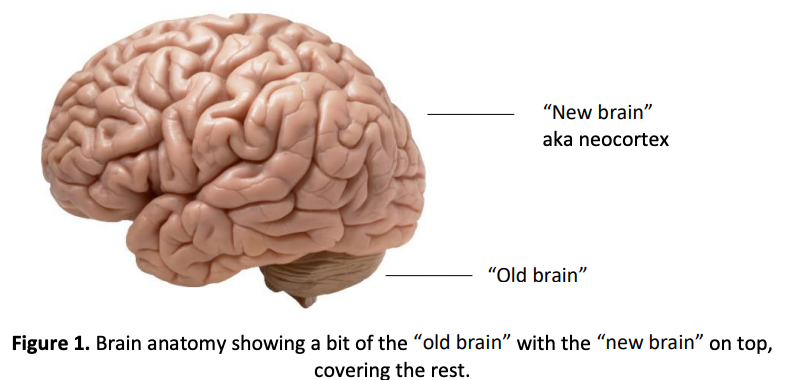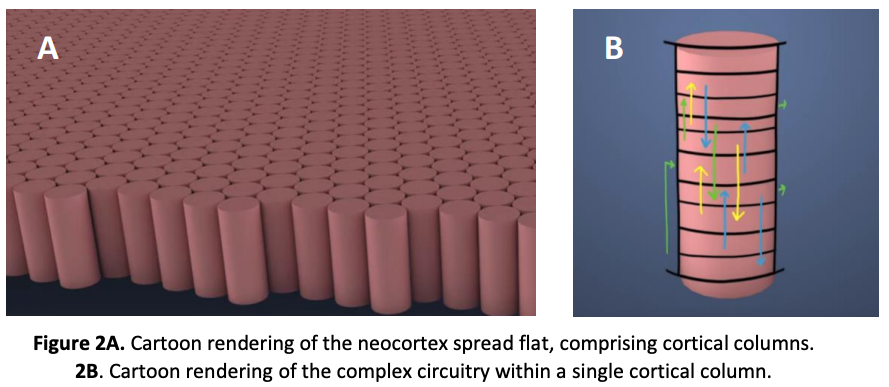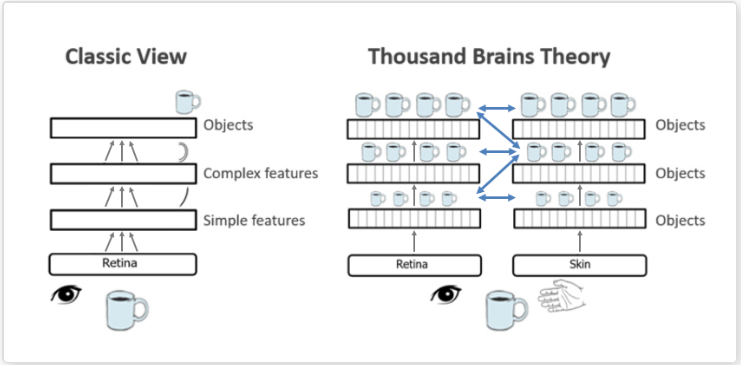Jeff 等人对现行的类神经网路，提出一个重要的质疑：类神经网路需要许多层以及大量已知的训练资料才能学习某些简单的任务，如辨认一个咖啡杯。但人类不需要看过千万张咖啡杯的照片才能学会什么是咖啡杯。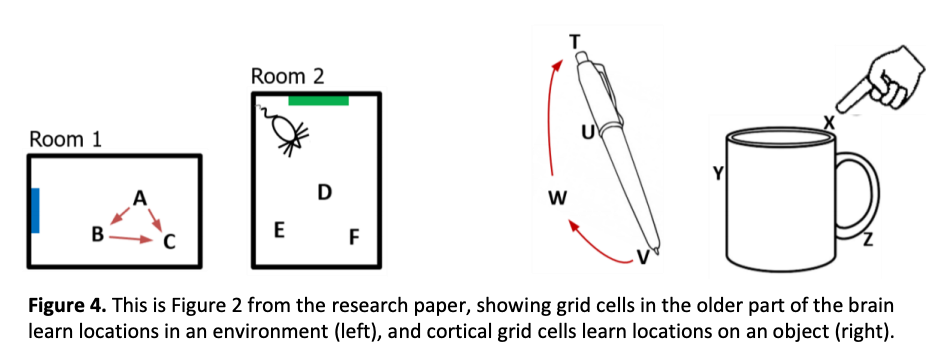1. 手指首先触碰到x点，学习到手指的触觉信号（杯口的感觉）与手指所在的位置（杯子上缘）。
2. 下一个时间点，手指移动到y点，同样学习到手指在y点的触觉（杯子的本体）以及手指在y点的位置（杯子左侧）。
3. 再下一个时间点，手指移动到z点，再次学习触觉信号（把手）以及位置讯号（杯子另一侧）
4. 根据以上三点，建立物件的轮廓（有圆圆的上缘、平直的一侧、有圆弧提把的另一侧等等），并以这个轮廓作为特征。
5. 下次手指碰触到同样的感觉序列的时候，人脑就知道这是个杯子。

1. 学习轮子看来的样子（习得：轮子）。
2. 学习窗户看起来的样子（习得：窗户）。
3. 学习引擎盖看起来的样子（习得：引擎盖）。
4. 学习车子看起来的样子（习得：车子＝轮子＋窗户＋引擎盖）。
5. 下次看到卡车，因为卡车也有同样的特征（轮子＋窗户＋引擎盖），故得知卡车也是一种车子，不用另外学习。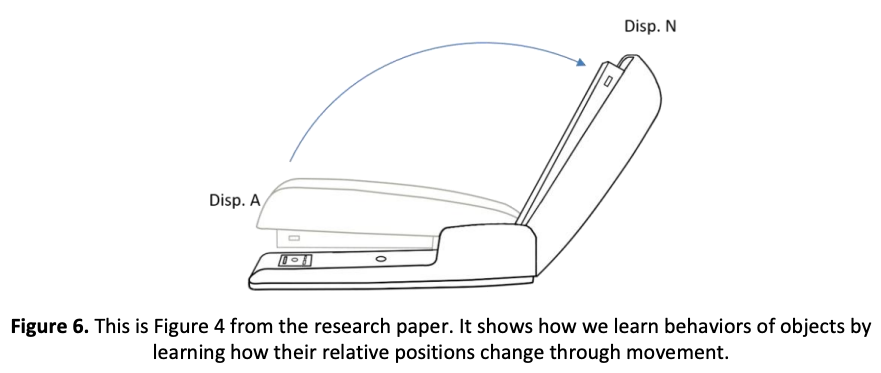1. 学习物体在 A位置的样子
2. 学习物体随着时间移动到 N的样子
3. 下次看到有一个物体有同样子的相对位置随时间变化的序列（钉书机打开— 闭合的序列）时，就说拥有这种「行为」的物体是钉书机。
4. 下次遇到一个被称为是「钉书机」的物体时，也会预期这个物体会展现同样的行为（打开— 闭合）。

Thousand Brain Theory of Intelligence理论的总整理：

• 千脑智能理论-因为每个皮质柱都可以学习完整的对象模型，所以大脑会同时创建数千个模型，而不是一个大模型。
• 位置，位置，位置-每个皮层柱都有一个位置信号，我们建议通过皮层网格单元来实现。
• 对象组成-我们提出了一种遍布大脑的新型神经元，即“置换细胞”，它们使我们能够了解对象是如何由其他对象组成的。
• 对象的行为-了解对象的行为只是简单地了解位移单元跟踪的运动顺序。
• 概念和高级思考-我们以学习物理对象的方式学习概念性想法。

## 具有皮质学习算法的HTM

HTM的层级结构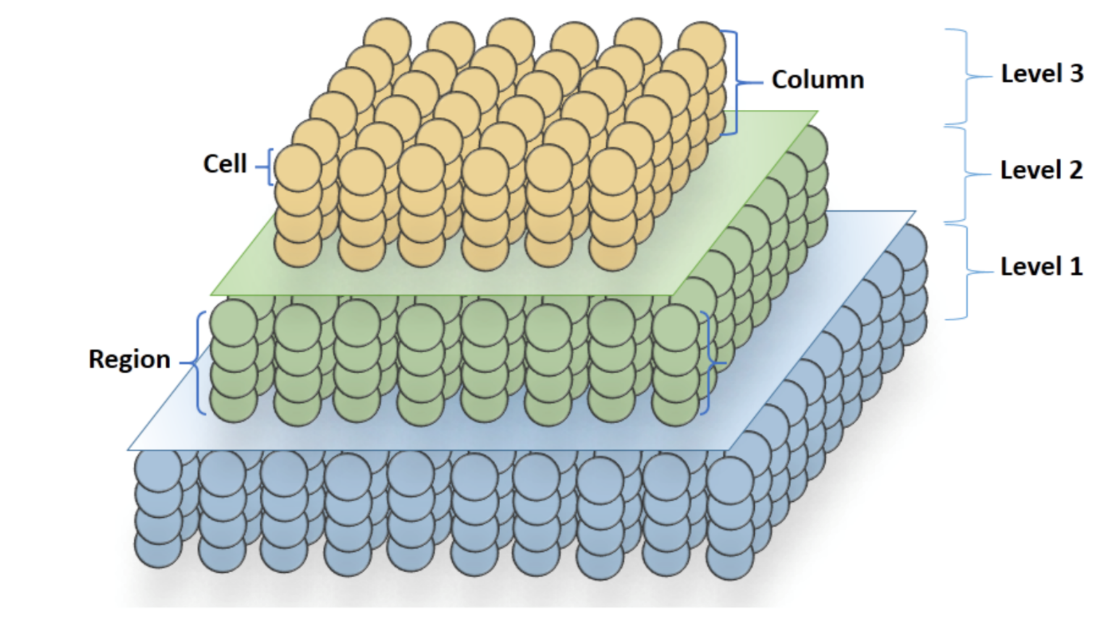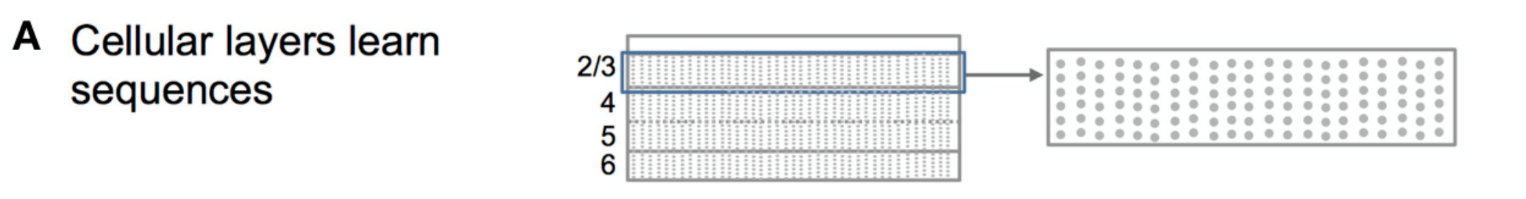HTM是多层的树状结构，感知器感知到的信号先输入到底层的节点，节点的输出（Beliefs）再层层传递到最高层。如下图：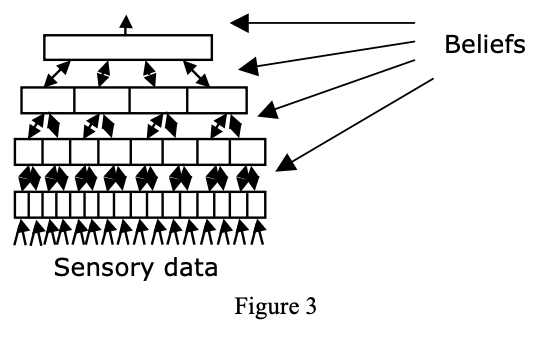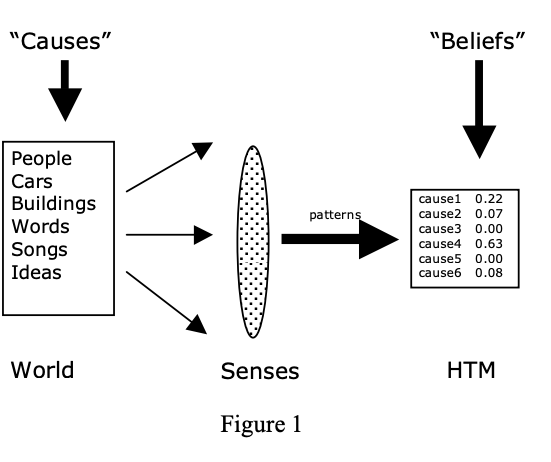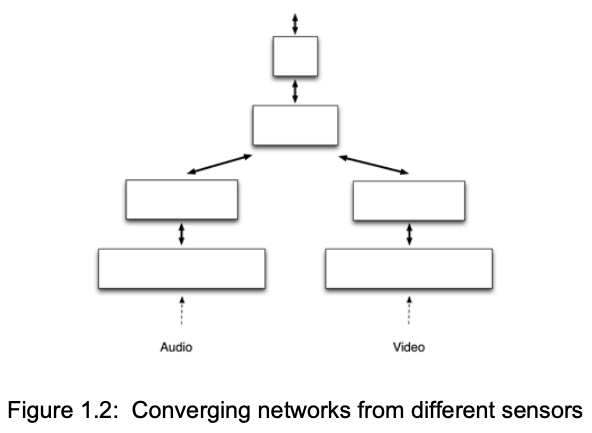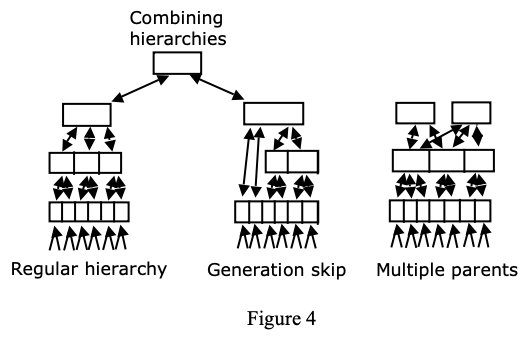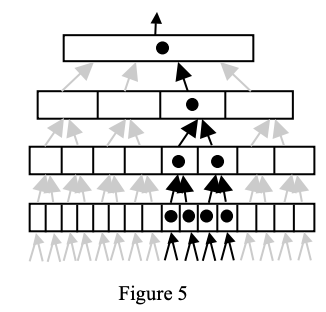HTM的Region可以理解为一个单一层，虽然我个人认为应该不需要特意限制一层只能有一个Region。Region的切面图如下图：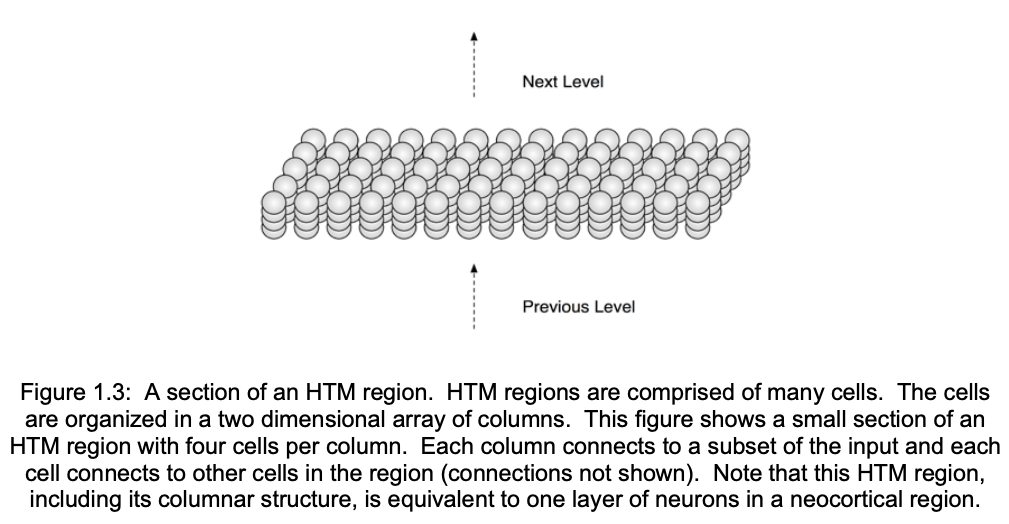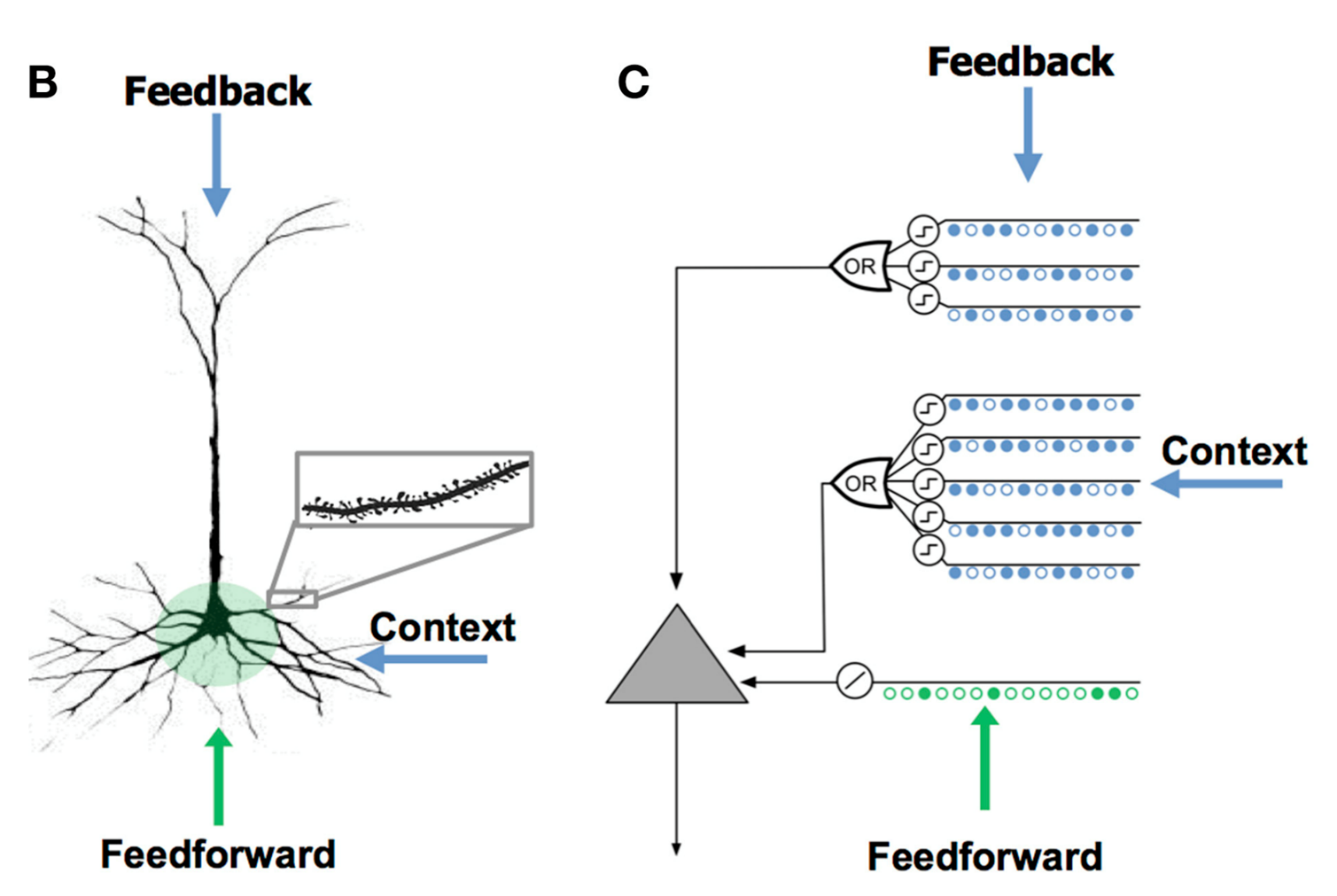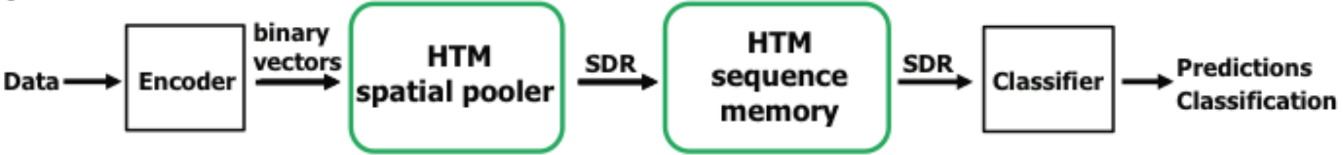HTM在实际应用过程中，一般会按照上图所示框架进行建模：原始数据进入数据编码器中，编码成二进制向量；然后再经HTM的空间池化（Spatial Pooling，SP)算法生成稀疏分布式代表元（Sparse Distributed Representation, SDR)；既而经HTM时间记忆（Temporal Memeory，TM）生成预测向量（也是SDR类型），最后将SDR输到分类器中进行分类预测，或其他函数、算法、模型中，实现所需目的。接下来，进行分块详述：数据编码（data encoding)、空间池化（spatial pooling）和时间记忆（temporal memeory）。

### 数据编码（data encoding)

HTM模拟新大脑皮质，而大脑皮质接收的数据对其功能有重要影响。神经科学已有很多研究表明感觉器官将接收到的信息转化成稀疏数据。如耳蜗上面的绒毛细胞：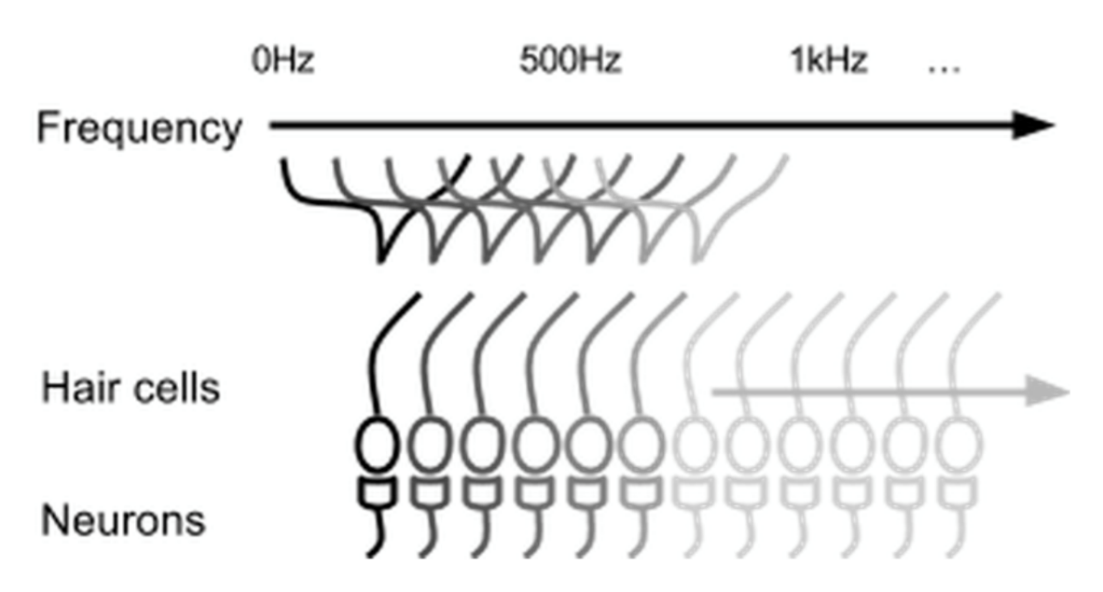• 语义相似数据应生成有重叠的激活位点（bits）；
• 相同输入总产生固定长度的二进制向量；
• 输出的二进制向量应有相同维度（位点总数）；
• 二进制向量应有相似的稀疏度，并且有足够的位点忍受噪音，以及子采样（subsampling)

Sparse distributed representations (SDR) 稀疏分布式代表元， 超大容量(tremendous capacity)，极度容忍噪声（extremely tolerant). SDR 对学习和记忆系统特别方便（之前的版本中，原始数据经编码过程产生的Output被命名为SDR,但最新版放弃了这种说法，转而把Spatial Pooling过程产生的output称为SDR；不过encoder产生的output也完全符合SDR性质，为叙述方便，不加区分，统称为SDR，至于代指哪一个过程，根据上下文过程可很容易区分）。输入到单元(cell)内的数据来自三种区域：

• 近端树突（proximal dendrites/zone):授受前馈输入，即接受原始数据经encoder产生的SDR, 定义最优感受野（classic receptive field)， 通常，同一个细胞列（柱）内分享同一近端树突；
• 远端树突(distal dendrites/basal zone)：接受背景环境输入,即接受同一区域（region）的SDR，也就是与同一层内的其它单元产生连接突触（注region一词指代不清，也因目前的算法发展层面，接下来假设每一层都只有一个区域，这种简化只是方便叙述，对理解，及对模型几乎没有影响。）,学习序列转换，即学习数据的时间结构；
• 顶端树突（apical dendrites/ apical zone):接受反馈输入，唤起自上而下的‘序列’期望。

1、Overlap: 用以衡量两个SDR相似性：

$$overlap(\boldsymbol{x,y}) = x \cdot y$$

$$match(x,y) \equiv overlap(x,y) \ge \theta$$

2、Union: 赋予HTM在一个固定长度代表元上存储大量模式的能力。这个能力使得HTM可以实时预测（在TM阶段)：

$$Union(x_1,x_2,\dots ) = \oplus(x_1,x_2,\dots)$$

### 空间池化（spatial pooling）

Spatial Pooling 是一种将输入讯号转变为Sparse Distributed Representation pattern的方法。“Pooling”这个字在论文中用来代指由输入讯号空间（较大空间）映射到Cortical region的sparse distributed representation空间（较小空间）所产生的、数学上的多对一映射问题。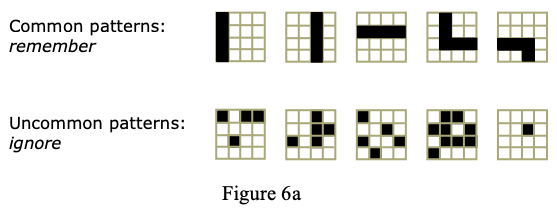• 在每个Column上找出输入造成的synapses overlap
• 找出被激发的candidate column，计算抑制半径
• update column的内部值

for c in columns :
overlap(c) = 0
for s in connectedSynapses(c):
overlap(c) = overlap(c) + input(t, s.sourceInput)
if overlap(c) < minOverlap:
overlap(c) = 0
else:
overlap(c) = overlap(c) * boost(c)


for c in columns:
minLocalActivity = kthScore(neighbors(c), desiredLocalActivity)
if overlap(c) > 0 and overlap(c) >= minLocalActivity:
activeColumns(t).append(c)


for c in activeColumns(t):
for s in potentialSynapses(c):
if active(s):
s.permanence += permanenceInc
s.permanence = min(1.0, s.permanence)
else:
s.permanence -= permanenceDec
s.permanence = max(0.0, s.permanence)
for c in columns:
minDutyCycle(c) = 0.01 * maxDutyCycle(neighbors(c))
activeDutyCycle(c) = updateActiveDutyCycle(c)
boost(c) = boostFunction(activeDutyCycle(c), minDutyCycle(c))

overlapDutyCycle(c) = updateOverlapDutyCycle(c)
if overlapDutyCycle(c) < minDutyCycle(c):
increasePermanences(c, 0.1*connectedPerm)


Spatial Pooler (SP):SP对前馈连接进行建模，负责将二进制输入数据转化成SDR。SP即是对数据的空间(spatial)信息进行提取。SP须满足的属性：

• fixed sparseness 固定稀疏性，即编码器出来的数据
• Distributed coding 分布式编码
• Preserving semantic similarity 保留语义相似性
• Noise robustness/ fault tolerance 噪声、错误的容忍
• Continuous learning 连续学习
• Stability 稳定性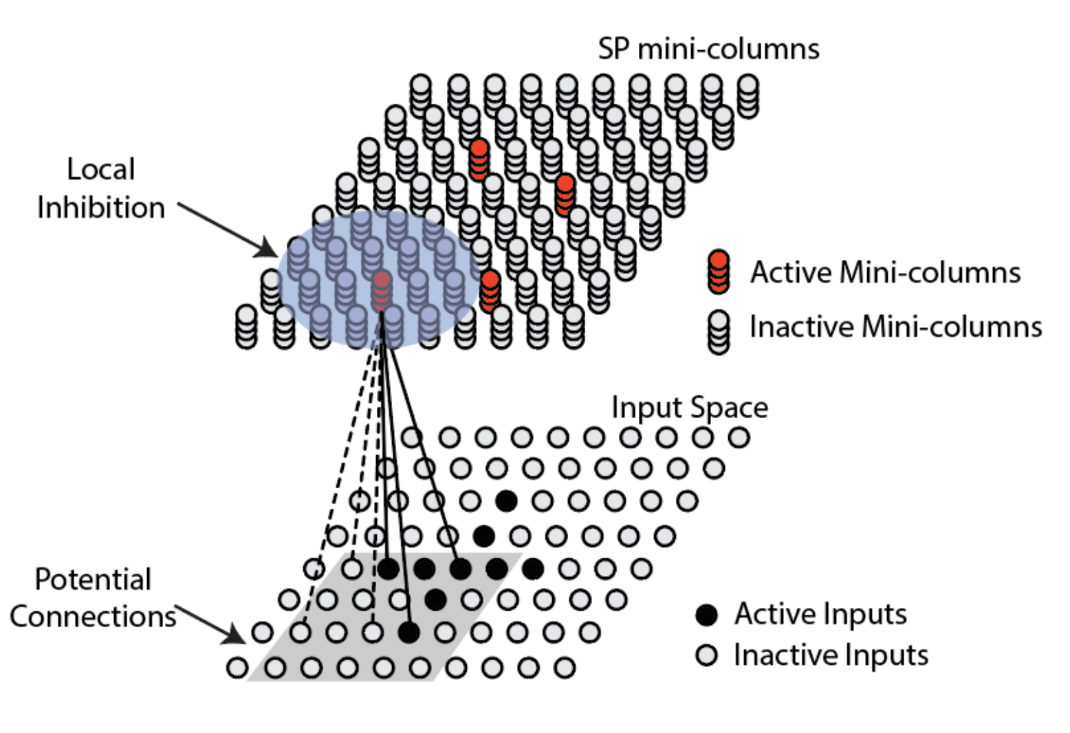SP模型的第一步是确定标准感受野（Classic Receptive Field）（上图中的灰色方框），即每个单元柱（mini-column)读取输入数据的区域，也就是说每个单元柱只读取输入数据的一部分，而且相邻单元柱的标准感受野是有重叠的(overlap)。标准感受野确定之后，则要确定其中的潜在突触连接（potential connection)。所谓潜在连接即为单元柱可以形成与数据形成连接突触：

$$PotentialInput(i) = \{j |I(x_j,x_i^c,\gamma)\ and \ Z_{ij} <p\}$$

$x_j$表示第j个输入数据（神经元）的位置，$x_i^c$表示标准感受野的中心输入神经元的位置。$\gamma$表示局部感受野的边长(矩形)；$Z_{ij} \sim U(0,1)$,即服从均匀分布的一个随机数; p 表示成为潜在连接的阈值，只有小于此阈值，才能成为潜在连接，即形成连接突触（此处暗示，标准感受野内的数据并是都会被SP读取，但目前从算法的代码上看，p值被设成1，即标准感受野内的数据都会成为潜在连接,故此处可以先不用考虑p值问题，下面提到的标准感受与潜在连接等同）。潜在连接成为突触连接，须突触的持久度(permanence) 不小于突触稳定阈值。持久度是形成突触连接的调节参数，是SP需要学习的参数，非常关键。初始化是随机为每个连接突触分配一个持久度。然后根据持久度域值，形成突触连接一个二进制矩阵WW(维度与标准感受野相同）:

$$W_{ij} = \left\{\begin{array}\\ 1, if \ D_{ij} \ge \theta_c\\ 0, otherwise \end{array}\right.$$

$$D_{ij} = \left\{\begin{array} \\ U(0,1), &\quad &j \in PotentialInput(i)\\ 0,&\quad& otherwise \end{array} \right.$$

$\theta_c$为持久阈值(例如：0.5).

$$o_i = b_i \sum_{j} W_{ij} z_j = b_i W_i Z$$

SP还对局部抑制(local inhibition)机制进行建模，也称’赢家通吃’原则,即，在第 i 个单元柱及其 n 个相邻单元柱中，如果第 i 个单元柱排在前k( k<n)个单元列中才会被激活。不过单元列的前馈输入的重合度(overlap) 还要不小于激活阈值($\theta_{stim}$)。用$a_i$表示第 i 个单元列的激活状态，则：

$$a_i = \left\{ \begin{array}\\ 1, \quad o_i \ge prctile(NeighborOverlap(i), 1 – s) \quad and\quad o_i \ge \theta_{stim};\\ 0, \quad otherwise. \end{array} \right.$$

$$NeighboringOverlap(i) = \{o_j| j \in Neighbor(i)\}$$

$$Neighbor(i) = \{j | \left\| y_i – y_j\right\| <\phi, j \ne i\}$$

$$\bar{a}_i(t) =\frac{ (T – 1) *\bar{a}_i(t-1) + a_i(t)}{T}$$

$$<\bar{a}_i(t)> = \frac{1}{|Neighbor(i)|} \sum_{j \in Neighbor(i)} \bar{a}_j(t)$$

$$b_i = exp(-\beta(\bar{a}_i(t) – <\bar{a}_i(t)>))$$

#### SP总结

• 经SP过程，产生真正意义上的SDR。SP过程，有多个步骤使数据稀疏化（当然这些步骤是在完成提取必要信息的过程中完成的稀疏化）：第一个是 潜在连接的产生过程（当然目前，算法的p值设为1，没有实际意义，但不应该忽略其作用）；第二个是根据持久度形成的突触连接；第三个是竞争性局部抑制。
• SP后，产生的SDR维度与这一层的维度一致（即在目前算法中，为一个M维向量，这里的M为本层内单元柱的个数）。
• SP激活的最小单位是单元柱，这也是前面强调的，同一单元柱内的单元共享近端树突的数据输入。其中提取的是数据 的空间特征。

## 时间记忆（Temporal Memeory）

Temporal Memory的基本概念是学习Spatial pattern的时间序列，如下图所示：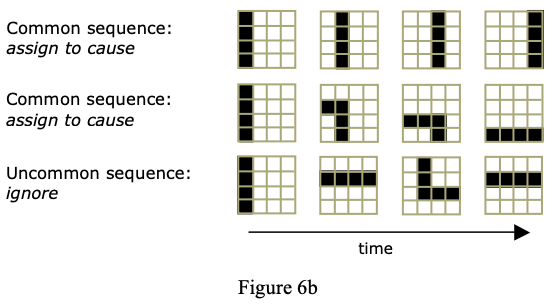TM 算法是HTM最核心的算法，正因为TM, HTM才具备的学习及预测能力。当SDR输入到TM中， 学习SDR中所代表的数据及其背景环境（context)。学习：通过增加或者减少潜在突触连接而达到学习目的。预测：根据SDR预测。基于过去与当前对未来进行预测。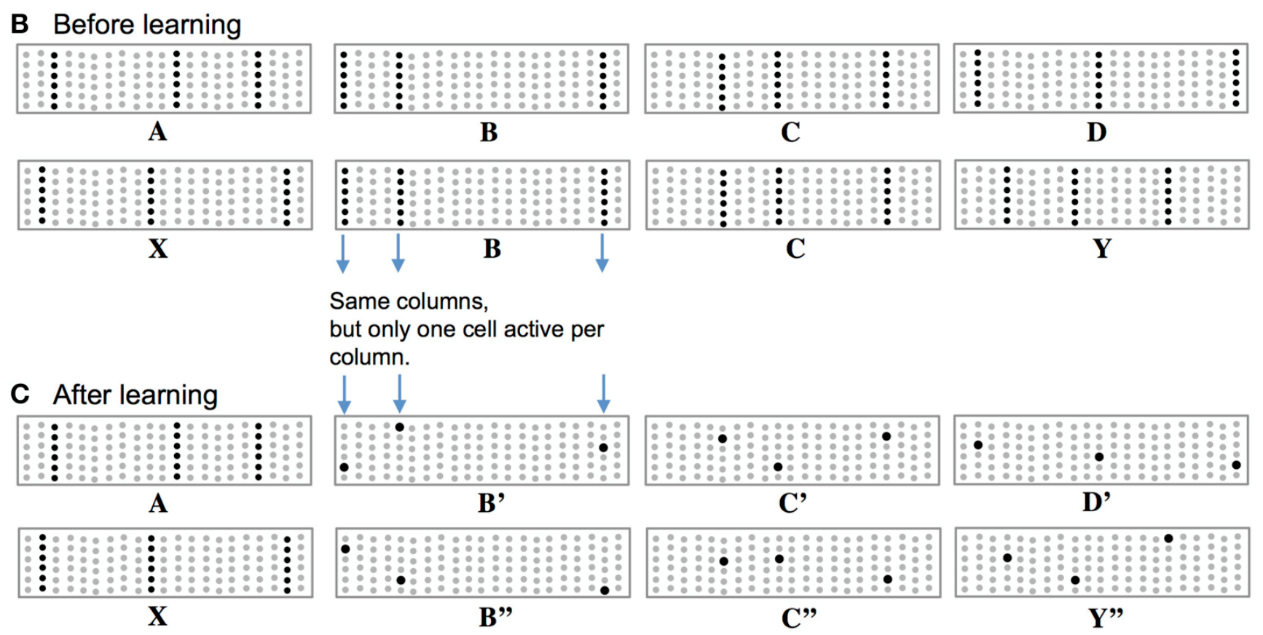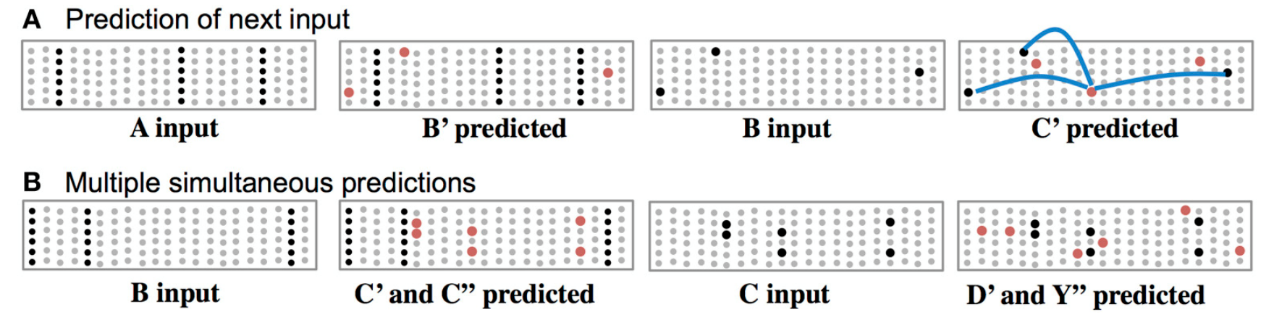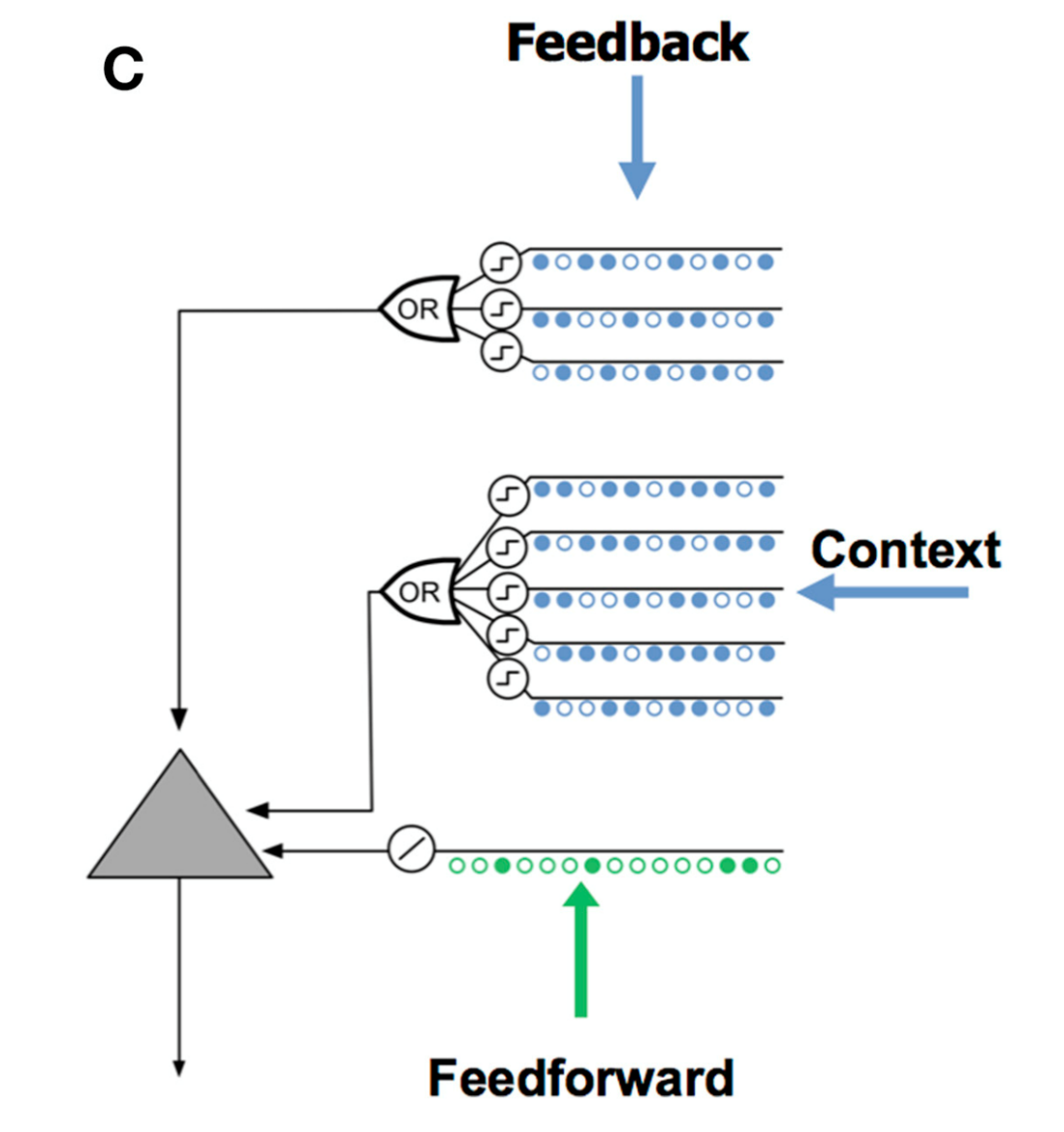• N: 单元柱数量
• M：每个单元柱所含单元数
• D: 每个单元所含基底树突数
• S：每个基底突触所突触数
• $A^t$：t时刻激活状态矩阵，其中$a_{ij}^t$表示在t时刻，第 j 个单元柱上第 i 个单元的状态
• $\Pi^t$：t时刻单元预测状态矩阵， 其中$\pi_{ij}^t$表示在t时刻，第 j 个单元柱上第 i 个单元的预测状态；
• $D_{ij}$：单元基底树突集，其中$D_{ij}^d$表示$a_{ij}^t$第j个单元柱上第i个单元的第 d 个基底树突，为一稀疏矩阵（MXN），其中非0元素代表，潜在突触的持久度
• $\dot{D}_{ij}^d$: MXN的0，1矩阵，其中元素1所处位置表示处此处的$D_{ij}$的持久度为非0(>0)
• $\tilde{D}_{ij}^d$: MXN 的0，1矩阵，其中元素1所处位置表示此处形成突触连接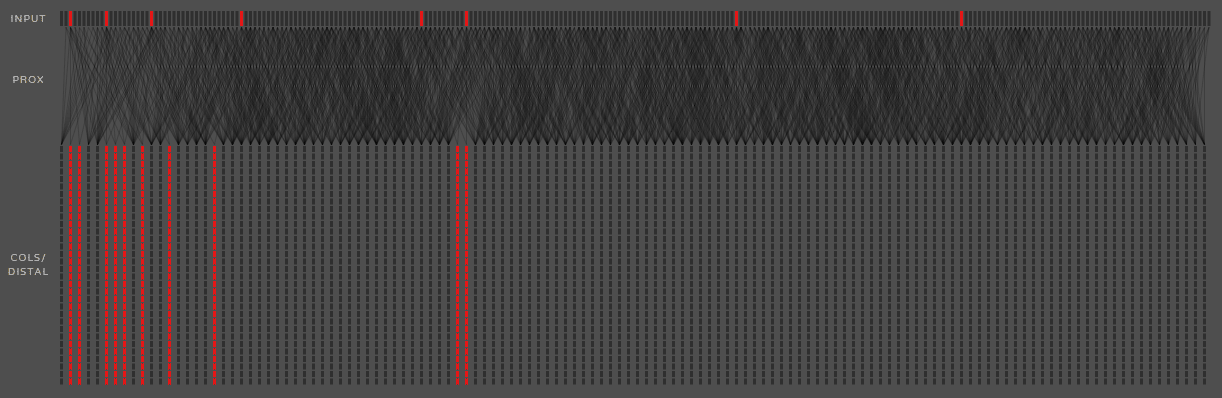$$a_{ij}^t = \left\{ \begin{array} \\ 1, \quad j\in W^t , \pi_{ij}^{t-1} = 1;\\ 1,\quad j \in W^t,\sum_{i} \pi_{ij}^{t-1} = 0;\\ 0,\quad otherwise. \end{array} \right.$$

$$\pi_{ij}^t = \left\{\begin{array} \\ 1, \quad \exists_d \left \| \tilde{D}_{ij}^d \circ A^t \right\|_1 > \theta;\\ 0,\quad otherwise. \end{array} \right.$$

1、更新树突选择

TM的学习及预测的物理基础即为树突的长衰，而树突的长衰则是通过树突的持久度来调节。那第一步即是选择要更新的树突。设要更新的树突集合为$D_u$,则：

$$D_{u1} :\left\{D_{ij}^d \ \Big|\ \ \forall_{j\in W^t}\left( \pi_{ij}^{t-1} > 0\right) and \left\| \tilde{D}_{ij}^d \circ A^{t-1}\right\| >\theta\right\}$$

$D_{u1}$表示那些成功预测的单元基底树突。

$$D_{u2} :\left\{D_{ij}^d \ \Big|\ \ \forall_{j\in W^t}\left( \sum_{i}\pi_{ij}^{t-1} = 0\right) and \left\| \dot{D}_{ij}^d \circ A^{t-1}\right\| = \max_{i}\left(\left\|\dot{D}_{ij}^d \circ A^{t-1}\right\|_1\right)\right\}$$

$$D_u = D_{u1} + D_{u2}$$

2、持久度更新

$$D_{ij}^d: =D_{ij}^d + \Delta D_{ij}^d$$

$$\Delta D_{ij}^d = \left\{ \begin{array} \\ p^+\left(\dot{D}_{ij}^d\circ A^{t-1}\right) – p^-\dot{D}_{ij}^d,&&\quad a_{ij}^t = 1;\\ p^{–}\dot{D}_{ij}^d,&&\quad a_{ij}^t = 0 \ and \ \left\| \tilde{D}_{ij}^d \circ A^{t-1}\right\| >\theta. \end{array} \right.$$

Inference Only — Phase 1:

for c in activeColumns(t):
buPredicted = false
for i = 0 to cellsPerColumn - 1 :
if predictiveState(c, i, t-1) == true:
s = getActiveSegment(c, i, t-1, activeState)
if s.sequenceSegment == true:
buPredicted = true
activeState(c, i, t) = 1
if buPredicted == false:
for i = 0 to cellsPerColumn - 1:
activeState(c, i, t) = 1


Inference Only — Phase 2

for c, i in cells:
for s in segments(c, i):
if segmentActive(c, i, s, t):
predictiveState(c, i, t) = 1


Inference + Learning — Phase 1

for c in activeColumns(t):
buPredicted = false
lcChosen = false
for i = 0 to cellsPerColumn - 1:
if predictiveState(c, i, t-1) == true:
s = getActiveSegment(c, i, t-1, activeState)
if s.sequenceSegment == true:
buPredicted = true
activeState(c, i, t) = 1
if segmentActive(s, t-1, learnState):
lcChosen = true
learnState(c, i, t) = 1
if buPredicted == false:
for i = 0 to cellsPerColumn - 1:
activeState(c, i, t) = 1
if lcChosen == false:
I,s = getBestMatchingCell(c, t-1)
learnState(c, i, t) = 1
sUpdate = getSegmentActiveSynapses (c, i, s, t-1, true)
sUpdate.sequenceSegment = true


Inference + Learning — Phase 2

for c, i in cells:
for s in segments(c, i):
if segmentActive(s, t, activeState):
predictiveState(c, i, t) = 1
activeUpdate = getSegmentActiveSynapses(c, i, s, t,
false)
predSegment = getBestMatchingSegment(c, i, t-1)
predUpdate = getSegmentActiveSynapses(c, i, predSegment,
t-1, true)


Inference + Learning — Phase 3

for c, i in cells:
if learnState(s, i, t) == 1:
segmentUpdateList(c, i).delete()
else if predictiveState(c, i, t) == 0
and predictiveState(c, i, t-1)==1:
segmentUpdateList(c, i).delete()


## 使用HTM进行时间序列异常检测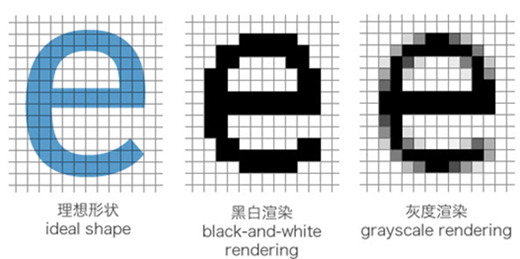##### 网站与APP开发中的字体设置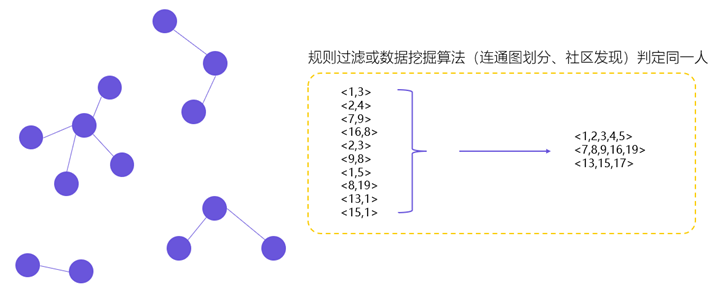##### 用户体系搭建之ID-Mapping